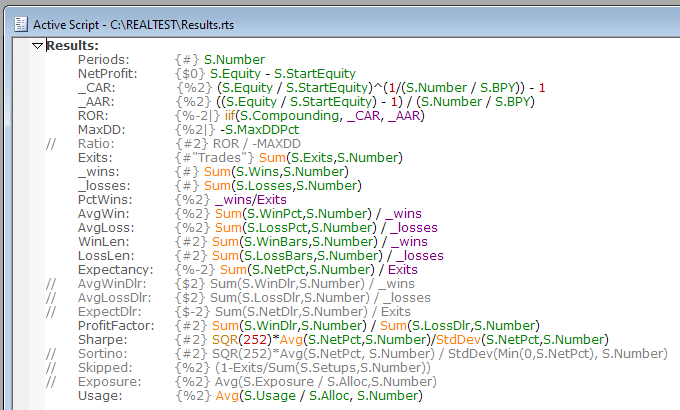Navigation: Realtest Script Language > Script Sections > Results Section

The Results Section is where the columns to display in any Results Window are defined.

Here is the default set of Results window columns and their definitions:You are welcome to edit this file (press F9 when viewing a results window) and/or include a different set of column definitions in other test scripts.

Examples of some columns that you might want to add are provided as comments in this default script (rows beginning with //).

Every test result record contains the same set of underlying Test Statistics Arrays (the green items in the above example). Your column formulas select which stats you want to display, allow you to specify the format of the numbers, and make it possible to calculate higher-level stats however you prefer.

Results column formulas are calculated only once, at the end of a test run. When they are calculated, the context is the last date of the test.

This is why, for example, S.Number is used to display the number of periods (dates) over which a test was run. The daily stats record for the first date is 1, the second date is 2, and so on. At the end of the above test, there were 6,894 stats records, so the number of the last record was 6,894.

Similarly, for stats where we want the total count of something for the entire test, we use the Sum function.

Results formulas can optionally reference Trade Statistics Functions, though calculating these can be slow for tests with very high trade counts.

A format specification comment can be included in any item. If no format is specified, the item will use default number formatting.

The vertical bar {|} in a Results item format specification indicates that this item should be calculated after each day of the test and displayed in the status bar as the test is running.

To apply Results section formulas to all currently open Results windows, press F4 or click Apply.